Rahul VikrantRohini Sector 16, Delhi, India - 110089Rahul VikrantTutor

Rohini Sector 16, Delhi, India - 110089.

3 Students taught

5.0

UrbanPro Rating

Details verified of Rahul Vikrant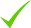IdentityEducation

Know how UrbanPro verifies Tutor details

Identity is verified based on matching the details uploaded by the Tutor with government databases.

Overview

I am an experienced, qualified teacher and tutor with over 4 years of experience in teaching Maths, across different boards including CBSE, ICSE and state boards. Passionate about solving Mathematical problems over the years I have helped thousands of students overcome their fear of Maths.

Languages Spoken

Hindi Mother Tongue (Native)

English Proficient

Education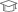BRABU, Muzaffarpur 2016

Bachelor of Science (B.Sc.)

Rohini Sector 16, Delhi, India - 110089

Verified Info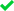ID VerifiedEducation VerifiedPhone VerifiedEmail VerifiedFacebook Verified

Demo Class

Yes, not charged

Report this Profile

Is this listing inaccurate or duplicate? Any other problem?

Please tell us about the problem and we will fix it.

Please describe the problem that you see in this page.

Type the letters as shown below *

Please enter the letters as show below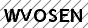Class 12 Tuition Overview

Class 12 Tuition

Class LocationStudent's HomeTutor's HomeOnline (video chat via skype, google hangout etc)

Years of Experience in Class 12 Tuition

5

Board

State, ISC/ICSE, CBSE

ISC/ICSE Subjects taught

Mathematics

CBSE Subjects taught

Mathematics

Taught in School or College

No

State Syllabus Subjects taught

Mathematics

Gallery (5)

Sample notes of Integration.

Sample notes of Integration.

Sample notes of Integration.

XI... complex numbers notes

XI... Complex number notes.

Reviews (1)

this is test message this is test message this is test message this is test message this is test message this is test message this is test message

5.0 out of 5.0 1 review

5.0051
N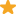"Fantastic teacher and friendt is really lucky to have a teacher like Rahul sir.Excellent knowledge about the subject. "

Thank you Nishchal.

Have you attended any class with Rahul? Write a Review

FAQs

1. Which school boards of of Class 11 do you teach for?

State, ISC/ICSE, CBSE

2. Have you ever taught in any School or College?

No

3. Which classes do you teach?

I teach Class 10 Tuition, Class 11 Tuition, Class 12 Tuition and Class 9 Tuition Classes.

4. Do you provide a demo class?

Yes, I provide a free demo class.

5. How many years of experience do you have?

I have been teaching for 5 years.

Answers by Rahul (1)

Answered on 17 Jul CBSE/Class 10/Mathematics/UNIT I: Number systems/Real numbers/NCERT Solutions/Exercise 1.1

Let 'a' be any +ve integer. Also suppose there exists two +ve integers q and r as quotient and remainder respectively when 'a' is divided by 6. Then , By using Euclid's Division lemma, we get a = 6q + r .................( A ) , where 0 ≤ r < 6. Obviously, r = 0 or 1 or 2 or 3 or... ...more

Let  'a' be any +ve integer.

Also suppose there exists two +ve integers q and r as quotient and remainder respectively when 'a' is divided by 6.

Then ,

By using Euclid's Division lemma, we get

a = 6q + r .................( A ) , where  0 ≤ r < 6.

Obviously,  r = 0 or 1 or 2 or 3 or 4 or 5.

Case-(i) When r = 0 , then from ( A ) we have

a = 6q + 0

=> a = 6q = 2×3q = 2m = Even number , where  m = 3q

Case-(ii) When r = 1 , then

a = 6q + 1 = 2(3q) + 1 = 2m + 1 = odd number, where m = 3q.

Case-(iii) When r = 2 then

a = 6q + 2 = 2(3q + 1) = 2m= Even , where m1 = 3q + 1.

Case-(iv) When r = 3 then

a = 6q + 3 = 2(3q + 1) + 1 = 2m1 + 1= odd number, where m= 3q + 1

Case-(v) When r= 4 then

a = 6q + 4 = 2(3q + 2) = 2m= Even , where m2 = 3q +2 .

Case -(vi) When r = 5 then

a = 6q + 5 = 2(3q + 2) + 1 = 2m+ 1 = odd number, where m= 3q + 2.

Clearly from above cases ( ii ) , ( iv ) and ( vi ) we conclude that any +ve odd integer is of the form 6q + 1 or 6q + 3 or 6q + 5.

* PROVED  *

Dislike Bookmark

RahulDirections

x
Class 12 Tuition 5.0

Class LocationStudent's HomeTutor's HomeOnline (video chat via skype, google hangout etc)

Years of Experience in Class 12 Tuition

5

Board

State, ISC/ICSE, CBSE

ISC/ICSE Subjects taught

Mathematics

CBSE Subjects taught

Mathematics

Taught in School or College

No

State Syllabus Subjects taught

Mathematics

Class 11 Tuition 5.0

Class LocationStudent's HomeTutor's HomeOnline (video chat via skype, google hangout etc)

Years of Experience in Class 11 Tuition

5

Board

State, ISC/ICSE, CBSE

ISC/ICSE Subjects taught

Mathematics

CBSE Subjects taught

Mathematics

Experience in School or College

I've experience of more than 5 years of teaching mathematics of 11 and 12.

Taught in School or College

Yes

State Syllabus Subjects taught

Mathematics

Class 10 Tuition 5.0

Class LocationStudent's HomeTutor's HomeOnline (video chat via skype, google hangout etc)

Years of Experience in Class 10 Tuition

5

Board

ICSE, CBSE, State

CBSE Subjects taught

Mathematics

ICSE Subjects taught

Mathematics

Taught in School or College

No

State Syllabus Subjects taught

Mathematics

Class 9 Tuition 5.0

Class LocationStudent's HomeTutor's HomeOnline (video chat via skype, google hangout etc)

Years of Experience in Class 9 Tuition

5

Board

ICSE, CBSE, State

CBSE Subjects taught

Mathematics

ICSE Subjects taught

Mathematics

Taught in School or College

No

State Syllabus Subjects taught

Mathematics

this is test message this is test message this is test message this is test message this is test message this is test message this is test message

5.0 out of 5.0 1 review

N"Fantastic teacher and friendt is really lucky to have a teacher like Rahul sir.Excellent knowledge about the subject. "

Thank you Nishchal.

Have you attended any class with Rahul? Write a Review

Answers by Rahul (1)

Answered on 17 Jul CBSE/Class 10/Mathematics/UNIT I: Number systems/Real numbers/NCERT Solutions/Exercise 1.1

Let 'a' be any +ve integer. Also suppose there exists two +ve integers q and r as quotient and remainder respectively when 'a' is divided by 6. Then , By using Euclid's Division lemma, we get a = 6q + r .................( A ) , where 0 ≤ r < 6. Obviously, r = 0 or 1 or 2 or 3 or... ...more

Let  'a' be any +ve integer.

Also suppose there exists two +ve integers q and r as quotient and remainder respectively when 'a' is divided by 6.

Then ,

By using Euclid's Division lemma, we get

a = 6q + r .................( A ) , where  0 ≤ r < 6.

Obviously,  r = 0 or 1 or 2 or 3 or 4 or 5.

Case-(i) When r = 0 , then from ( A ) we have

a = 6q + 0

=> a = 6q = 2×3q = 2m = Even number , where  m = 3q

Case-(ii) When r = 1 , then

a = 6q + 1 = 2(3q) + 1 = 2m + 1 = odd number, where m = 3q.

Case-(iii) When r = 2 then

a = 6q + 2 = 2(3q + 1) = 2m= Even , where m1 = 3q + 1.

Case-(iv) When r = 3 then

a = 6q + 3 = 2(3q + 1) + 1 = 2m1 + 1= odd number, where m= 3q + 1

Case-(v) When r= 4 then

a = 6q + 4 = 2(3q + 2) = 2m= Even , where m2 = 3q +2 .

Case -(vi) When r = 5 then

a = 6q + 5 = 2(3q + 2) + 1 = 2m+ 1 = odd number, where m= 3q + 2.

Clearly from above cases ( ii ) , ( iv ) and ( vi ) we conclude that any +ve odd integer is of the form 6q + 1 or 6q + 3 or 6q + 5.

* PROVED  *

Dislike Bookmark

Rahul Vikrant describes himself as Tutor. He conducts classes in Class 10 Tuition, Class 11 Tuition and Class 12 Tuition. Rahul is located in Rohini Sector 16, Delhi. Rahul takes at students Home, Regular Classes- at his Home and Online Classes- via online medium. He has 5 years of teaching experience . Rahul has completed Bachelor of Science (B.Sc.) from BRABU ,Muzaffarpur in 2016. He is well versed in Hindi and English. Rahul has got 1 reviews till now with 100% positive feedback.

Recommended Profiles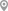Rohini Sector 16, DelhiRohini Sector 16, DelhiRohini Sector 16, DelhiRohini Sector 16, DelhiRohini Sector 16, DelhiRohini Sector 16, Delhi

X

Post your Learning Need

Let us shortlist and give the best tutors and institutes.

or

Send Enquiry to Rahul

Let Rahul know you are interested in their class

Reply to 's review